|

# 壁纸选得好 能省下一大笔钱

壁纸属于墙面装饰中款式和颜色比较多的一种，是美感和逼格的必备神器!多彩的款式犹如一幅幅大师级的艺术作品，在墙面巨大的画板上，演绎万种风情。为完成壁纸创作的“大作”，咱们先好好了解种类、选购、保养的那些事吧~

1、壁纸的分类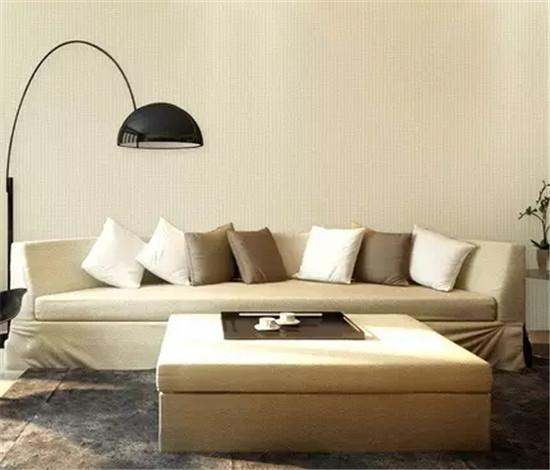硅藻土壁纸

以硅藻土为原料，表面有无数细孔，可吸附、分解空气中的异味，具有调湿、除臭、隔热、防止细菌生长等功能，其有助于净化室内空气。

推荐场所：居室、书房、客厅、办公地点、衣柜等。

无纺布壁纸

以纯无纺布为基材，表面采用水性油墨印刷后涂上特殊材料，经特殊加工而成，具有吸音、不变形等优点、。因为其非常地薄，施工起来非常容易，非常适合喜欢DIY的年轻人。

推荐人群：年轻一族。

纯纸类壁纸

以纸为基材，经印花后压花而成，自然、舒适、无异味、环保性好，透气性能强。纸质不好的产品时间久了可能会略显泛黄。

推荐场所：儿童房等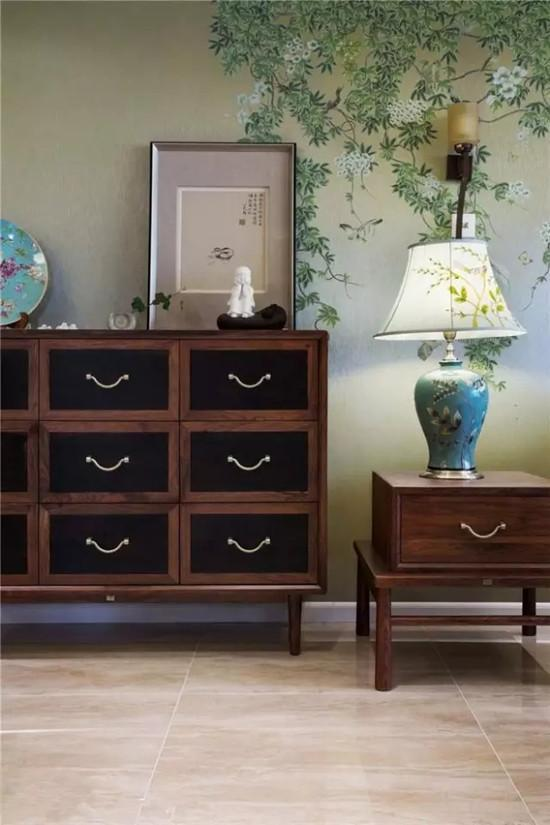树脂类壁纸

面层用胶来构成，也叫高分子材料。这类壁纸防水性能非常的好，水分不会渗透到墙体里面去，属于隔离型防水。

推荐场所：公共区位、洗手间等。

织物类壁纸

以丝绸、麻、棉等编织物为原材料，其物理性状非常地稳定，湿水后颜色变化也不大，在市场上也是非常地受欢迎，但相比无纺布类，价格较高。

推荐人群：消费能力较高者、喜欢自然气息者。

墙布类壁纸

其特点主要是面层相对厚重，其给人感觉结实耐用。要注意的是不宜使用在卧室等休息的场所，以免让人压抑紧张。

推荐场合：高档装修设计等。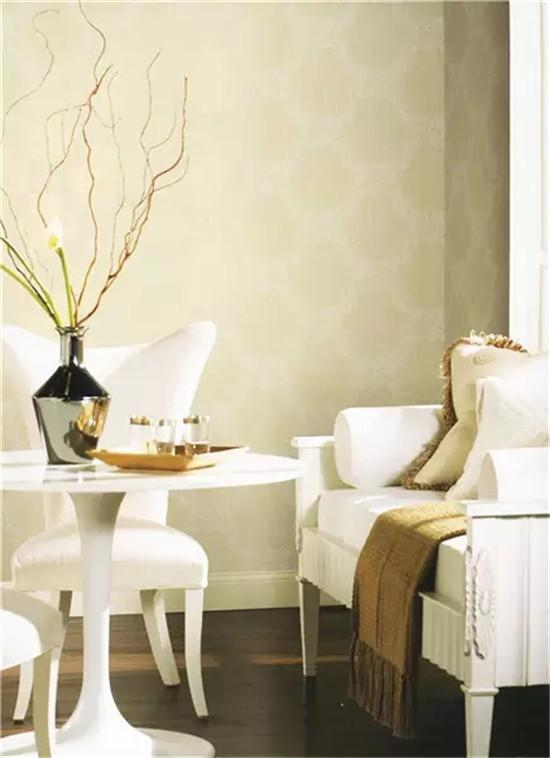云母片类壁纸

云母是一种硅酸盐结晶，因此这类产品高雅有光泽感，加上很好的电绝缘性，相对来说导电性弱，安全系数高，既美观又实用，有小孩的家庭非常喜爱。

推荐场所：公众场所、沙发背景、客厅电视背景墙等。

天然材料类壁纸

由天然的草木加工而成，自然古朴，具有保暖通风、吸音防潮的功能，给人带来返璞归真的体验。不足的是由于材料天然，铺装时接缝明显，不能精细平整。

推荐人群：喜欢自然古朴者。

金银箔类壁纸

主要采用纯正的金、银、丝等高级面料制成表层，手工工艺精湛，贴金工艺考究，价值较高，并且防水防火，易于保养。

推荐人群：消费能力极强者。

和纸类壁纸

和纸被称为“纸中之王”，与中国的宣纸有点像，非常地耐用，几千年颜色和物理形状都不会发生变化。其给人以清新脱俗之感，并兼具防水防火性能，不过大多价格昂贵。

推荐人群：消费能力较高者、品味高雅者。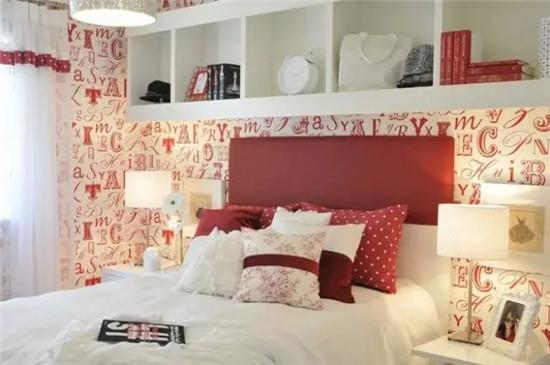2、壁纸的挑选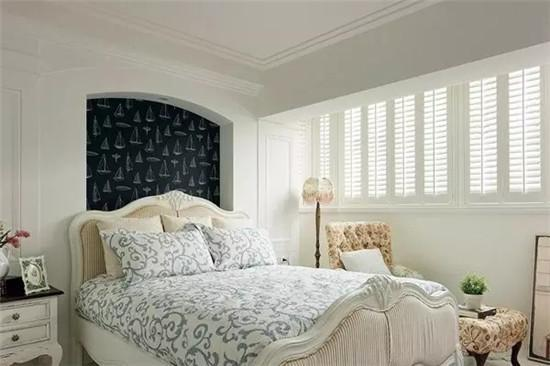挑选比之前做好规划，按需选购

在挑选比之前规划好那些地方需要贴壁纸，壁纸的风格、颜色、图案、特性等，什么样的空间用什么样的壁纸。在整体风格统一的前提下，各空间的壁纸挑选可根据他的功能来定，然后在尽心购买计划，减少壁纸浪费。

按壁纸铺贴面积合理购买，减少浪费

壁纸适合使用在卧室房间或客厅的四面墙壁，根据预算不同，选择全贴壁纸或者背景墙部分贴壁纸，如整面铺会比小面积要暗，需要考虑整张效果。

壁纸用量计算：国产纸的尺寸是0.53m*10m，韩国纸的尺寸是1.06m*15.6m。

所需壁纸=墙宽/墙纸规格*可裁剪条数。

在实际粘贴中，壁纸存在8%左右的合理损耗，大花壁纸的损耗更大，因此在采购时应留出消耗量，这样可以做到适量而不浪费。合理规划壁纸辅料，全面节省

贴壁纸需要的辅料包括基膜(刷在墙面上，有防霉、防潮、抗碱的作用)、胶粉(掺水搅拌好之后，刷在壁纸背面的)、胶浆(一种粘合剂，一般刷在壁纸的两侧边缘，起收边的作用)。

在挑选壁纸时，也要了解一下他们的使用功能、质量，建议选择拥有成套系统的品牌，配套使用不仅效果好，而且全面兼节省，可以防止不熟悉而乱购买。3、壁纸的保养

壁纸发霉绝大多数的原因，并不是出在壁纸本身上头，而是出在墙体结构的工程品质。由于墙体品质不良，容易发生渗水问题，如果刚好又是挑选到透气性差的壁纸材质，那么就有相当大的机会出现「霉点」。

挑选透气材料

市面上的壁纸材质琳瑯满目，有不织布、PVC、木纤维、玻璃纤维等等，其中又以PVC材质最不透气，容易将墙体所排出的水气闷在里头，久而久之就容易产生霉点。

确保墙面干燥

在水泥完工后，一般须保养20天至40天，让墙面达到完全乾燥，才能进行壁纸的铺贴。也要特别注意是否有海沙混入，因为海沙含盐量高，容易吸聚水分。当然也要将壁面缺口填平，以免造成空鼓。注意铺贴品质

俗话说：「三分料七分工」，再好的材质没有纯熟的施工品质也是枉然。铺贴时除了要注意拼花是否对齐之外，还要准确拿捏收缩缝的预留宽度，也要尽量避免收缩缝开口直接对向迎风口。

妥善维护保养

不论是在施工期间，或者是完工后的24小时，尽量不要将门窗全开，因为过大的对流风势会造成壁纸急剧干燥，产生收缩不匀的状况。而在完工后则是要注意调节通风，防止潮气进入影响黏贴的牢固程度。

4、壁纸赏析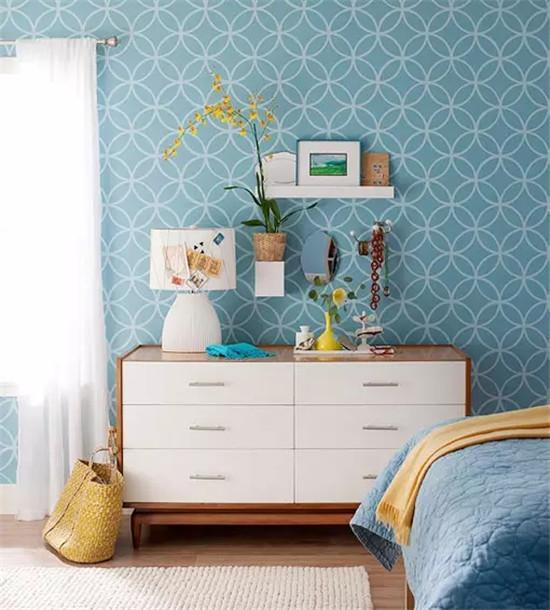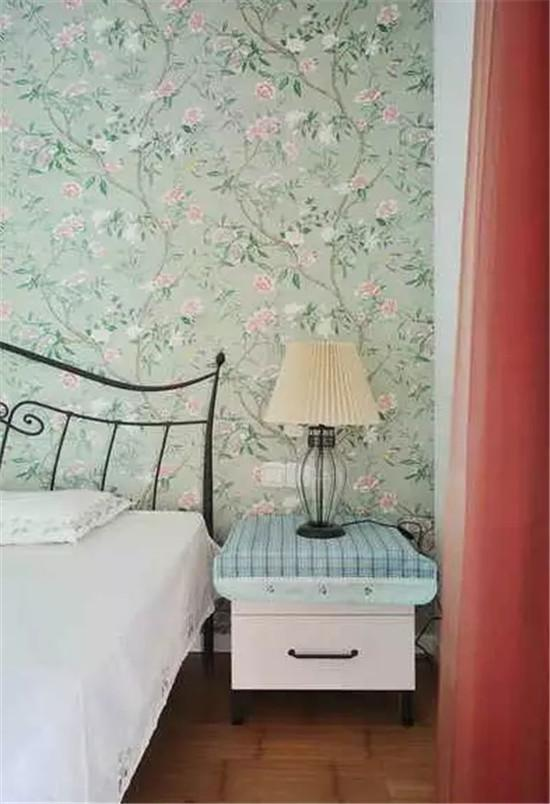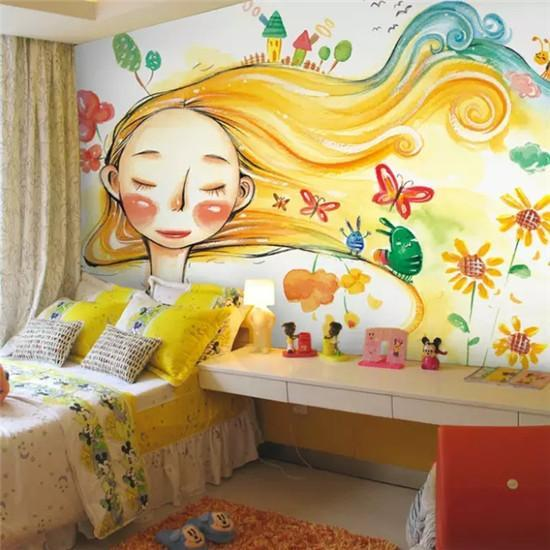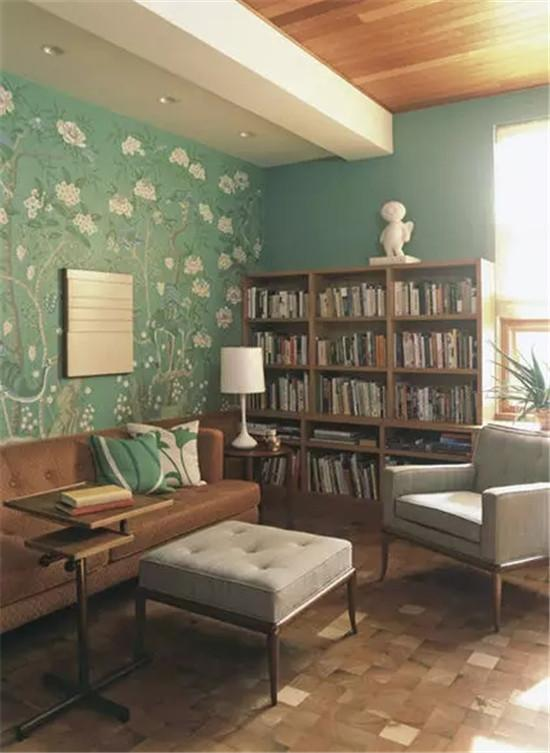`声明：本文由入驻焦点开放平台的作者撰写，除焦点官方账号外，观点仅代表作者本人，不代表焦点立场错误信息举报电话： 400-099-0099，邮箱：jubao@vip.sohu.com，或点此进行意见反馈，或点此进行举报投诉。`A B C D E F G H J K L M N P Q R S T W X Y Z
A - B - C - D - E
• A
• 鞍山
• 安庆
• 安阳
• 安顺
• 安康
• 澳门
• B
• 北京
• 保定
• 包头
• 巴彦淖尔
• 本溪
• 蚌埠
• 亳州
• 滨州
• 北海
• 百色
• 巴中
• 毕节
• 保山
• 宝鸡
• 白银
• 巴州
• C
• 承德
• 沧州
• 长治
• 赤峰
• 朝阳
• 长春
• 常州
• 滁州
• 池州
• 长沙
• 常德
• 郴州
• 潮州
• 崇左
• 重庆
• 成都
• 楚雄
• 昌都
• 慈溪
• 常熟
• D
• 大同
• 大连
• 丹东
• 大庆
• 东营
• 德州
• 东莞
• 德阳
• 达州
• 大理
• 德宏
• 定西
• 儋州
• 东平
• E
• 鄂尔多斯
• 鄂州
• 恩施
F - G - H - I - J
• F
• 抚顺
• 阜新
• 阜阳
• 福州
• 抚州
• 佛山
• 防城港
• G
• 赣州
• 广州
• 桂林
• 贵港
• 广元
• 广安
• 贵阳
• 固原
• H
• 邯郸
• 衡水
• 呼和浩特
• 呼伦贝尔
• 葫芦岛
• 哈尔滨
• 黑河
• 淮安
• 杭州
• 湖州
• 合肥
• 淮南
• 淮北
• 黄山
• 菏泽
• 鹤壁
• 黄石
• 黄冈
• 衡阳
• 怀化
• 惠州
• 河源
• 贺州
• 河池
• 海口
• 红河
• 汉中
• 海东
• 怀来
• I
• J
• 晋中
• 锦州
• 吉林
• 鸡西
• 佳木斯
• 嘉兴
• 金华
• 景德镇
• 九江
• 吉安
• 济南
• 济宁
• 焦作
• 荆门
• 荆州
• 江门
• 揭阳
• 金昌
• 酒泉
• 嘉峪关
K - L - M - N - P
• K
• 开封
• 昆明
• 昆山
• L
• 廊坊
• 临汾
• 辽阳
• 连云港
• 丽水
• 六安
• 龙岩
• 莱芜
• 临沂
• 聊城
• 洛阳
• 漯河
• 娄底
• 柳州
• 来宾
• 泸州
• 乐山
• 六盘水
• 丽江
• 临沧
• 拉萨
• 林芝
• 兰州
• 陇南
• M
• 牡丹江
• 马鞍山
• 茂名
• 梅州
• 绵阳
• 眉山
• N
• 南京
• 南通
• 宁波
• 南平
• 宁德
• 南昌
• 南阳
• 南宁
• 内江
• 南充
• P
• 盘锦
• 莆田
• 平顶山
• 濮阳
• 攀枝花
• 普洱
• 平凉
Q - R - S - T - W
• Q
• 秦皇岛
• 齐齐哈尔
• 衢州
• 泉州
• 青岛
• 清远
• 钦州
• 黔南
• 曲靖
• 庆阳
• R
• 日照
• 日喀则
• S
• 石家庄
• 沈阳
• 双鸭山
• 绥化
• 上海
• 苏州
• 宿迁
• 绍兴
• 宿州
• 三明
• 上饶
• 三门峡
• 商丘
• 十堰
• 随州
• 邵阳
• 韶关
• 深圳
• 汕头
• 汕尾
• 三亚
• 三沙
• 遂宁
• 山南
• 商洛
• 石嘴山
• T
• 天津
• 唐山
• 太原
• 通辽
• 铁岭
• 泰州
• 台州
• 铜陵
• 泰安
• 铜仁
• 铜川
• 天水
• 天门
• W
• 乌海
• 乌兰察布
• 无锡
• 温州
• 芜湖
• 潍坊
• 威海
• 武汉
• 梧州
• 渭南
• 武威
• 吴忠
• 乌鲁木齐
X - Y - Z
• X
• 邢台
• 徐州
• 宣城
• 厦门
• 新乡
• 许昌
• 信阳
• 襄阳
• 孝感
• 咸宁
• 湘潭
• 湘西
• 西双版纳
• 西安
• 咸阳
• 西宁
• 仙桃
• 西昌
• Y
• 运城
• 营口
• 盐城
• 扬州
• 鹰潭
• 宜春
• 烟台
• 宜昌
• 岳阳
• 益阳
• 永州
• 阳江
• 云浮
• 玉林
• 宜宾
• 雅安
• 玉溪
• 延安
• 榆林
• 银川
• Z
• 张家口
• 镇江
• 舟山
• 漳州
• 淄博
• 枣庄
• 郑州
• 周口
• 驻马店
• 株洲
• 张家界
• 珠海
• 湛江
• 肇庆
• 中山
• 自贡
• 资阳
• 遵义
• 昭通
• 张掖
• 中卫

1室1厅1厨1卫1阳台

1
2
3
4
5

0
1
2

1

1

0
1
2
3报名成功，资料已提交审核A B C D E F G H J K L M N P Q R S T W X Y Z
A - B - C - D - E
• A
• 鞍山
• 安庆
• 安阳
• 安顺
• 安康
• 澳门
• B
• 北京
• 保定
• 包头
• 巴彦淖尔
• 本溪
• 蚌埠
• 亳州
• 滨州
• 北海
• 百色
• 巴中
• 毕节
• 保山
• 宝鸡
• 白银
• 巴州
• C
• 承德
• 沧州
• 长治
• 赤峰
• 朝阳
• 长春
• 常州
• 滁州
• 池州
• 长沙
• 常德
• 郴州
• 潮州
• 崇左
• 重庆
• 成都
• 楚雄
• 昌都
• 慈溪
• 常熟
• D
• 大同
• 大连
• 丹东
• 大庆
• 东营
• 德州
• 东莞
• 德阳
• 达州
• 大理
• 德宏
• 定西
• 儋州
• 东平
• E
• 鄂尔多斯
• 鄂州
• 恩施
F - G - H - I - J
• F
• 抚顺
• 阜新
• 阜阳
• 福州
• 抚州
• 佛山
• 防城港
• G
• 赣州
• 广州
• 桂林
• 贵港
• 广元
• 广安
• 贵阳
• 固原
• H
• 邯郸
• 衡水
• 呼和浩特
• 呼伦贝尔
• 葫芦岛
• 哈尔滨
• 黑河
• 淮安
• 杭州
• 湖州
• 合肥
• 淮南
• 淮北
• 黄山
• 菏泽
• 鹤壁
• 黄石
• 黄冈
• 衡阳
• 怀化
• 惠州
• 河源
• 贺州
• 河池
• 海口
• 红河
• 汉中
• 海东
• 怀来
• I
• J
• 晋中
• 锦州
• 吉林
• 鸡西
• 佳木斯
• 嘉兴
• 金华
• 景德镇
• 九江
• 吉安
• 济南
• 济宁
• 焦作
• 荆门
• 荆州
• 江门
• 揭阳
• 金昌
• 酒泉
• 嘉峪关
K - L - M - N - P
• K
• 开封
• 昆明
• 昆山
• L
• 廊坊
• 临汾
• 辽阳
• 连云港
• 丽水
• 六安
• 龙岩
• 莱芜
• 临沂
• 聊城
• 洛阳
• 漯河
• 娄底
• 柳州
• 来宾
• 泸州
• 乐山
• 六盘水
• 丽江
• 临沧
• 拉萨
• 林芝
• 兰州
• 陇南
• M
• 牡丹江
• 马鞍山
• 茂名
• 梅州
• 绵阳
• 眉山
• N
• 南京
• 南通
• 宁波
• 南平
• 宁德
• 南昌
• 南阳
• 南宁
• 内江
• 南充
• P
• 盘锦
• 莆田
• 平顶山
• 濮阳
• 攀枝花
• 普洱
• 平凉
Q - R - S - T - W
• Q
• 秦皇岛
• 齐齐哈尔
• 衢州
• 泉州
• 青岛
• 清远
• 钦州
• 黔南
• 曲靖
• 庆阳
• R
• 日照
• 日喀则
• S
• 石家庄
• 沈阳
• 双鸭山
• 绥化
• 上海
• 苏州
• 宿迁
• 绍兴
• 宿州
• 三明
• 上饶
• 三门峡
• 商丘
• 十堰
• 随州
• 邵阳
• 韶关
• 深圳
• 汕头
• 汕尾
• 三亚
• 三沙
• 遂宁
• 山南
• 商洛
• 石嘴山
• T
• 天津
• 唐山
• 太原
• 通辽
• 铁岭
• 泰州
• 台州
• 铜陵
• 泰安
• 铜仁
• 铜川
• 天水
• 天门
• W
• 乌海
• 乌兰察布
• 无锡
• 温州
• 芜湖
• 潍坊
• 威海
• 武汉
• 梧州
• 渭南
• 武威
• 吴忠
• 乌鲁木齐
X - Y - Z
• X
• 邢台
• 徐州
• 宣城
• 厦门
• 新乡
• 许昌
• 信阳
• 襄阳
• 孝感
• 咸宁
• 湘潭
• 湘西
• 西双版纳
• 西安
• 咸阳
• 西宁
• 仙桃
• 西昌
• Y
• 运城
• 营口
• 盐城
• 扬州
• 鹰潭
• 宜春
• 烟台
• 宜昌
• 岳阳
• 益阳
• 永州
• 阳江
• 云浮
• 玉林
• 宜宾
• 雅安
• 玉溪
• 延安
• 榆林
• 银川
• Z
• 张家口
• 镇江
• 舟山
• 漳州
• 淄博
• 枣庄
• 郑州
• 周口
• 驻马店
• 株洲
• 张家界
• 珠海
• 湛江
• 肇庆
• 中山
• 自贡
• 资阳
• 遵义
• 昭通
• 张掖
• 中卫• 手机• 分享
• 设计
免费设计
• 计算器
装修计算器
• 入驻
合作入驻
• 联系
联系我们
• 置顶
返回顶部